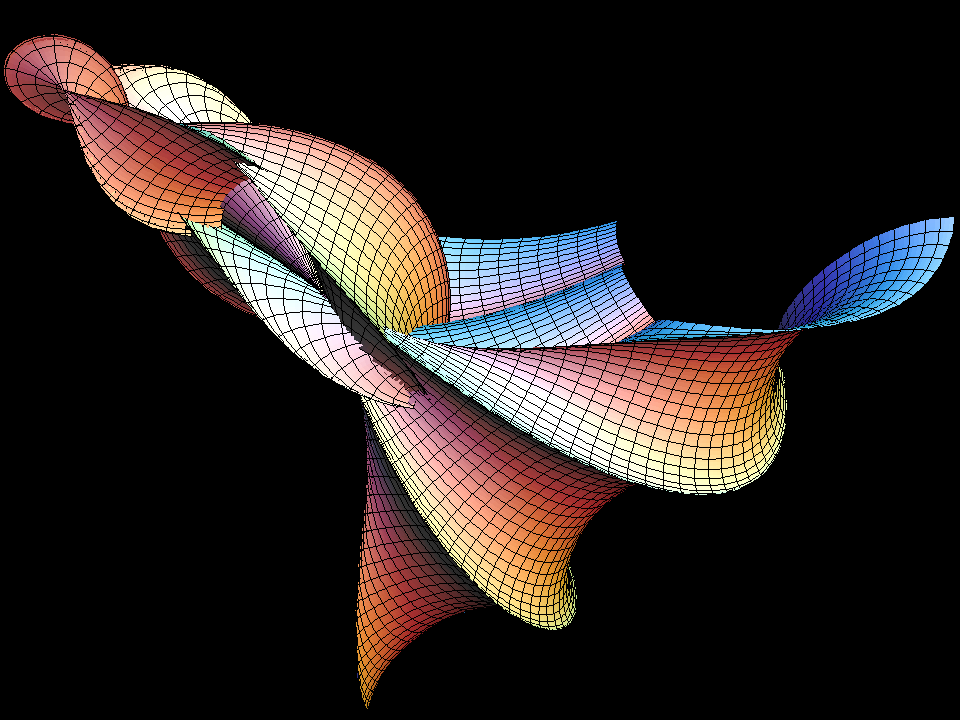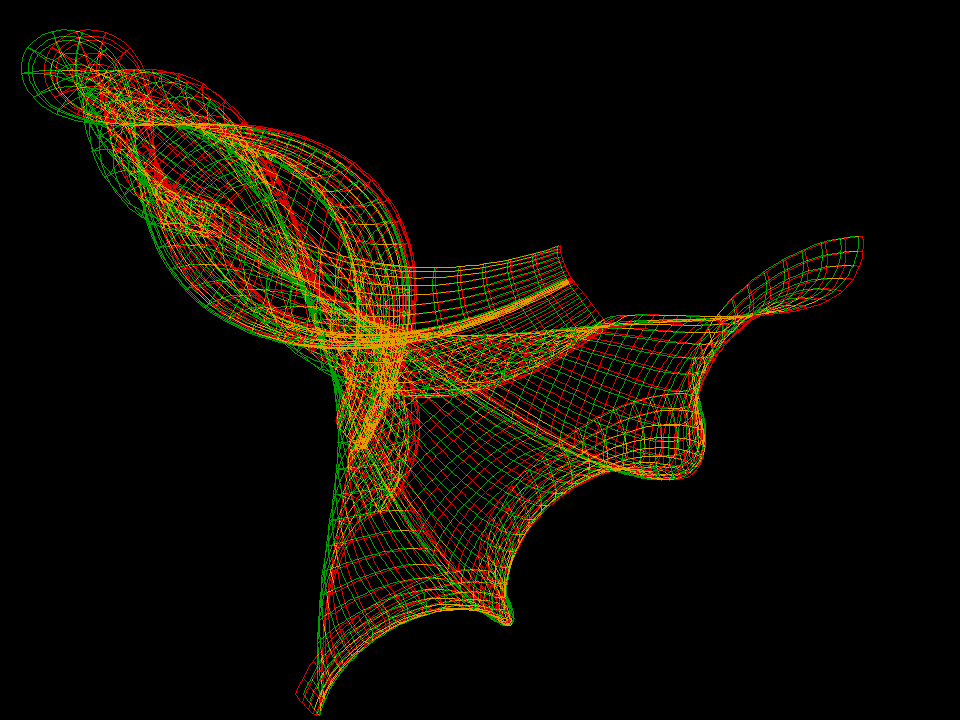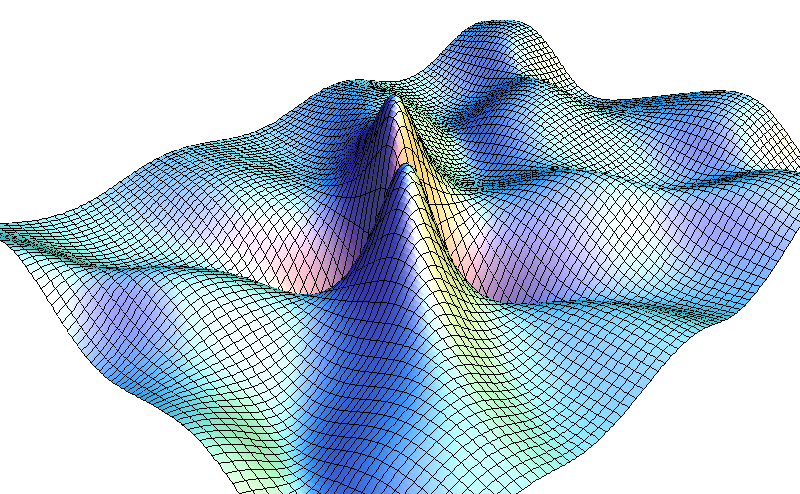# Four-Soliton Surface

The Bianchi-Bäcklund transform leads to more and more complicated, but explicit, solutions q(u,v) of the Sine-Gordon Equation (SGE). Each SGE solution determines a parametrized surface `F(u,v)` with Gauss curvature `K = -1` .

In spite of the sharp rims these surfaces are defined for all `(u,v)` in R^2. The singular rims occur in a similar way as the cusps of rolling curves: At the zeros of `sin(q(u,v))` the velocity of one set of parameter lines becomes zero and the parameter lines reverse their direction, so that each produces a cusp and all these cusps are a rim.Piece of a (K = -1) surface made from a 4-soliton SGE solution. The 4-soliton q(u,v) depends on four real constants a,b,c,d.Anaglyph stereo version of the surface above.Piece of a (K = -1) surface made from a 4-soliton SGE solution. The 4-soliton q(u,v) depends on two complex constants z=a+i*b , w=c+i*d , but still is a real SGE solution.The soliton q(u,v) of the previous surface is shown as the graph (u,v,q(u,v)) . This solution is called double breather.